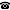# 2011 seminar talk: Positive Horn definability in \aleph_0-categorical structures

Talk held by Moritz Müller (KGRC) at the KGRC seminar on 2011-10-27.

### Abstract

We show that in an $$\aleph_0$$-categorical structure $$A$$ a relation is positive Horn definable if and only if it is preserved by the surjective periomorphisms of $$A$$. These are homomorphisms from the periodic power of $$A$$ into $$A$$, and the periodic power of $$A$$ is the structure induced on all periodic sequences in $$A^\omega$$.

It follows that the complexity (up to polynomial time reducibility) of the problem to decide the positive Horn theory of some $$\aleph_0$$-categorical structure is determined by the structures set of surjective periomorphisms.

Such problems are known as quantified constraint satisfaction problems and have been studied in depth for finite structures - there a preservation theorem as above has been established via surjective polymorphisms.

The talk gives some background information concerning the so-called algebraic approach to constraint complexity which is based on such preservation theorems.

This is joint work with Hubie Chen.

Kurt Gödel Research Center for Mathematical Logic. Währinger Straße 25, 1090 Wien, Austria.+43-1-4277-50501. Last updated: 2010-12-16, 04:37.